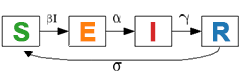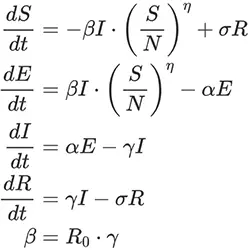# SEIR Model Calculator

This calculator offers a visualization of the SEIR (Susceptible-Exposed-Infected-Removed) epidemic model in graphic form.

SEIR | SEIRS Model Calculator

days

daysResults

## Equations

This model is described by the following equations:Where:

N = S + E + I + R  is the total population size,

S, E, I, R  denote the proportions of susceptible, exposed, infected, and recovered individuals respectively.